# HSSlive: Plus One & Plus Two Notes & Solutions for Kerala State Board

## BSEB Class 10 Maths Chapter 6 Triangles Ex 6.5 Textbook Solutions PDF: Download Bihar Board STD 10th Maths Chapter 6 Triangles Ex 6.5 Book AnswersBSEB Class 10 Maths Chapter 6 Triangles Ex 6.5 Textbook Solutions PDF: Download Bihar Board STD 10th Maths Chapter 6 Triangles Ex 6.5 Book Answers

BSEB Class 10th Maths Chapter 6 Triangles Ex 6.5 Textbooks Solutions and answers for students are now available in pdf format. Bihar Board Class 10th Maths Chapter 6 Triangles Ex 6.5 Book answers and solutions are one of the most important study materials for any student. The Bihar Board Class 10th Maths Chapter 6 Triangles Ex 6.5 books are published by the Bihar Board Publishers. These Bihar Board Class 10th Maths Chapter 6 Triangles Ex 6.5 textbooks are prepared by a group of expert faculty members. Students can download these BSEB STD 10th Maths Chapter 6 Triangles Ex 6.5 book solutions pdf online from this page.

## Bihar Board Class 10th Maths Chapter 6 Triangles Ex 6.5 Books Solutions

 Board BSEB Materials Textbook Solutions/Guide Format DOC/PDF Class 10th Subject Maths Chapter 6 Triangles Ex 6.5 Chapters All Provider Hsslive

## How to download Bihar Board Class 10th Maths Chapter 6 Triangles Ex 6.5 Textbook Solutions Answers PDF Online?

2. Click on the Bihar Board Class 10th Maths Chapter 6 Triangles Ex 6.5 Answers.
3. Look for your Bihar Board STD 10th Maths Chapter 6 Triangles Ex 6.5 Textbooks PDF.
4. Now download or read the Bihar Board Class 10th Maths Chapter 6 Triangles Ex 6.5 Textbook Solutions for PDF Free.

Find below the list of all BSEB Class 10th Maths Chapter 6 Triangles Ex 6.5 Textbook Solutions for PDF’s for you to download and prepare for the upcoming exams:

## BSEB Bihar Board Class 10th Maths Solutions Chapter 6 Triangles Ex 6.5

Question 1.
Sides of triangles are given below. Determine which of them are right triangles. In case of a right triangle, write the length of its hypotenuse.
(i) 7 cm, 24 cm, 25 cm
(ii) 3 cm, 8 cm, 6 cm
(iii) 50 cm, 80 cm, 100 cm
(iv) 13 cm, 12 cm, 5 cm
Solution:
(i) Let a = 7 cm, b = 24 cm and c = 25 cm.
Here the largest side is c = 25 cm.
We have : a² + b² = 7² + 24² = 49 + 576 = 625 = c²
So, the triangle with the given sides is a right triangle. Its hypotenuse is 25 cm.

(ii) Let a – 3 cm, 6 = 8 cm and c = 6 cm.
Here, the largest side is 6= 8 cm.
We have : a² + c² = 3² + 6² = 9 + 36 = 45 ≠ b²
So, the triangle with the given sides is not a right triangle.

(iii) Let a = 50 cm, b – 80 cm and c = 100 cm.
Here, the largest side is c = 100 cm.
We have : a² + b² = 50² + 80² = 2500 + 6400
= 8900 ≠ c²
So, the triangle with the given sides is not a right triangle.

(iv) Let a = 13 cm, b – 12 cm and c = 5 cm.
Here, the largest side is a = 13 cm.
We have : b² + c² = 12² + 5² = 144 + 25 = 169 = a²
So, the triangle with the given sides is a right triangle. Its hypotenuse is 13 cm.

Question 2.
PQR is a triangle right angled at P and M is a point on QR such that PM ⊥ QR. Show that PM² = QM.MR.
Solution:
Given : PQR is a triangle right angled at P and PM ⊥ QR.
To Prove : PM² = QM.MR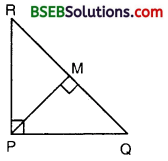Proof: Since PM ⊥ QR, therefore
∆ PQM ~ ∆ RPM
or 𝑃𝑀𝑄𝑀 = 𝑀𝑅𝑃𝑀
So, PM² = QM.MR.

Question 3.
In the figure, ABD is triangle right angled at and AC ⊥ BD. Show that
(i) Ab² = BC.BD
(ii) Ac² = BC.DCSolution:
Given : ABD is a triangle right angled at A and AC ⊥ BD.
To Prove :
(i) Ab² = BC.BD
(ii) Ac² = BC.DC
Proof:
(i) Since AC ⊥ BD, therefore
∆ ABC – ∆ DAC and each triangle is similar to the whole ∆ ABD.
∵ ∆ ABC – ∆ DBA
∴ 𝐴𝐵𝐷𝐵 = 𝐵𝐶𝐴𝐵 or AB² = BC.BD AB

(ii) Since ∆ ABC ~ ∆ DAC, therefore
𝐴𝐶𝐵𝐶 = 𝐷𝐶𝐴𝐶 or Ac² = BC.DC AC

(iii) Since ∆ ACD ~ ∆ BAD, therefore
∴ 𝐴𝐷𝐶𝐷 = 𝐵𝐷𝐴𝐷 or AD² = BD. CD.

Question 4.
ABC is an isosceles triangle right angled at C. Prove that Ab² = 2Ac².
Solution:
Since ABC is an isosceles A right triangle, right angled at C, therefore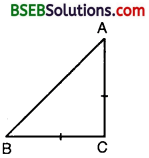Ab² = Ac² + Bc²
or Ab² = Ac² + Ac² [∵ BC = AC, given]
So, Ab² = 2 Ac²

Question 5.
ABC is an isosceles triangle with AC = BC. If Ab² = 2Ac², prove that ABC is a right triangle.
Solution:
Since ABC is an isosceles triangle with AC = BC and Ab² = 2Ac², therefore
Ab² = Ac² + Ac²
or Ab² = Ac² + Bc² [∵ AC = BC, given]
∴ ∆ ABC is right angled at C.

Question 6.
ABC is an equilateral triangle of side 2a. Find each of its altitudes.
Solution:
Let ABC be an equilateral triangle of side 2a units. Draw AD ⊥ BC. Then, D is the mid-point of BCSo, BD = 12 BC
= 12 x 2a = a
Since ABD is a right triangle, right angled at D, therefore
or (2a)² = AD² + (a)²
or AD² = 4a² – a² = 3a² or AD = 3‾√a
∴ Each of its altitudes is V3a.

Question 7.
Prove that the sum of the squares of the sides of a rhombus is equal to the sum o diagonals.
Solution:
Let the diagonals AC and BD of a rhombus ABCD intersect each other at O. Since the diagonals of a rhombus bisect each other at right angles.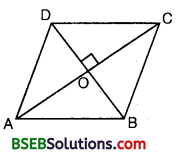∠AOB = ∠BOC – ∠COD
= ∠DOA = 90°
and AO = CO, BO = OD
Since AOB is a right triangle right
AB² = Oa² + Ob²
or AB² = (12AC)² +(12BD)² [∵ OA = OC and OB = OD]
or 4AB² = Ac² + BD² … (1)
Similarly, we have :
4BC² = Ac² + BD² …(2)
4CD² = Ac² + BD² …(3)
and 4AD² = Ac² + BD²
Adding all these results, we get
4(AB² + BC² + CD² + AD)² = 4(AC² + BD²)
or AB² + BC² + CD² + DA² = AC² + BD².

Question 8.
In the figure, O is a point in the interior of a triangle ABC, OD ± BC, OE ⊥ AC and OF ⊥ AB. Show that
(i) Oa² + Ob² + Oc² – OD² – OE² – OF² B = AF² + BD² + CE²
(ii) AF² + BD² + CE² = AE² + CD² + BF².Solution:
Join AO, BO and CO.(i) In right As OFA, ODB and OEC, we have :
OA² = AF² + OF²
Ob² = BD² + OD²
and OC² = CE² + OE²
OA² + OB² + OC² = AF² + BD² + CE² + OF² + OD² + OE² or OA² + OB² + OC² – OD² – OE² – OF² = AF² + BD² + CE².

(ii) In-right As ODB and ODC, we have :
OB² = OD² + BD²
and OC² = OD² + CD²
or OB² – OC² – BD² – CD² … (1)
Similarly, we have :
OC² – OA² = CE² – AE² … (2)
and OA² – OB² = AF² – BF² … (3)
Adding equations (1), (2) and (3), we get
(OB² – OC²) + (OC² – OA²) + (OA² – OB²)
= (BD² – CD²) + (CE² – AE²) + (AF² – BF²) or (BD² + CE² + AF²) – (AE² + CD² + BF²) = 0
or AF² + BD² + CE²
= AE² + BF2 + CD².

Aliter for (ii) :
Taking triangles OFB, ODC and OAE, we have :
OA² + OB² + OC²- OD²- OE² – OF² = AE² + BF² + CD²
So, using result (i) above, we have :
AF² + BD² + CE² = AE² + BF² + CD²

Question 9.
A ladder 10 m long reaches a window 8 m above the ground. Find the distance of the foot of the ladder from the base of the wall.
Solution:
Let AB be the ladder, B be the B window and CB be the wall. Then, ABC is a right triangle, right angled at C.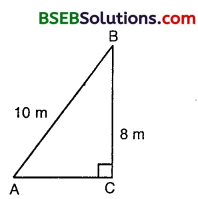∴ AB² = AC² + Bc²
So, 10² = AC² + 8²
or AC² = 100 – 64
or AC²= 36
or AC = 6 .A C
Hence, the foot of the ladder is at a distance of 6 m from the base of the wall.

Question 10.
A guy wire attached to a vertical pole of height 18 m is 24 m long and has a stake attached to the other end. How far from the base of the pole should the stake be driven so that the wire will be taut ?
Solution:
Let AB (= 24 m) be a guy wire attached to a vertical pole BC of height 18 m. To keep the wire taut, let it be fixed to a stake at A. Then, ABC is a right triangle, right angled at C.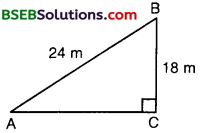∴ AB² = AC²+ Bc²
So, 24² = AC²+ 182
or AC² = 576 – 324
or AC² = 552
or AC = 252‾‾‾‾√ = 67‾√
Hence, the stake may be placed at a distance of 67‾√ m from the base of the pole.

Question 11.
An aeroplane leaves an airport and flies due north at a speed of 1000 km per hour. At the same time, another aeroplane leaves the same airport and flies due west at a speed of 1200 km per hour. How far apart will be the two planes after 1 12 hours?
Solution:
Let the first aeroplane starts from O and goes upto A towards north, where OA = (1000 x 32) km = 1500 km.Let the second aeroplane starts from O at the same time and goes upto B towards west, where OB = (1200 x 32) km = 1800 km.
According to the problem, the required distance = BA.
∆In right angled ∆ ABC, by Pythagoras theorem, we have :
AB² = OA² + OB²
= (1500)² + (1800)² = 2250000 + 3240000
= 5490000 = 9 x 61 x 100 x 100
or AB = 3 x 100 61‾‾‾√ km = 300 61‾‾‾√ km.

Question 12.
Two poles of heights 6 m and 11 m stand on a plane ground. If the distance between the feet of the poles is 12 m, find the distance between their tops.
Solution:Let AB = 11 m and CD = 6 m be the two poles such that BD = 12 m.
Draw CE ⊥ AB and join AC.
∴CE = DB = 12 m,
AE = AB – BE
= AB – CD
= (11 – 6) m = 5 m.
In right angled ∆ ACE, by Pythagoras theorem, we have :
AC² = CE² + AE²
= (12)² + (5)² = 144 + 25 = 169
So, AC = 169‾‾‾‾√ = 13
Hence, the distance between the tops of the two poles is 13 m.

Question 13.
D and E are points on the sides CA and CB respectively of a triangle ABC right angled at C. Prove that AE² + BD² = AB² + DE².
Solution:
In right angled ∆s ACE and DCB, we have :AE² = AC²+ CE²
and BD² = DC²+ Bc²
So, AE² + BD² = (AC²+ CE²) + (DC²+ Bc²)
AE² + BD² = (AC²+ Bc²) + (DC²+ CE²)
AE² + BD² = AB² + DE² [By Pythagoras theorem, AC²+ BC²= AB² and DC²+ CE² = DE²]

Question 14.
The perpendicular from A on side BC of a ∆ ABC intersects BC at D such that DB = 3 CD (see figure). Prove that 2AB² = 2AC²+ BC².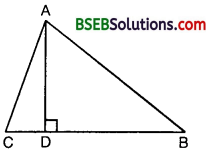Solution:
We have : DB = 3CD
Now, BC = DB + CD
i.e., BC = 3CD + CD [∵ BD = 3CD]
or BC = 4CD
∴ CD = 14 BC and DB = 3CD = 34 BC … (1)
Since ∆ ABD is a right triangle, right angled at D, therefore by Pythagoras theorem, we have :
AB² = AB² + DB²
Similarly, from ∆ACD, we have :
(1) – (2) givesQuestion 15.
In an equilateral triangle ABC, D is a point on side BC such that BD = 13 BC. Prove that 9AD² = 7AB².
Solution:
Let ABC be an equilateral triangle and let D be a point on BC such that
BD = 13BC.
Draw AE ⊥ BC. Join AD.In ∆s AEB and AEC, we have :
AB = AC [∴∆ABC is equilateral]
∠AEB = ∠AEC [∵ Each = 90°]
and AE = AE
∴ By SAS criterion of congruence, we have :
∆ AEB = ∆ AEC
So, BE = EC
Now, we have :
BD = 13BC, DC = 23BC
BE = EC = 12BC … (1)
Since ∠C = 60°, therefore triangle.
∆ ADC is an acute triangle.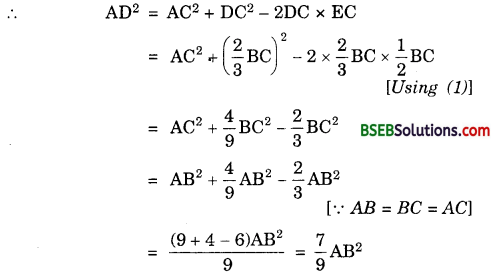So, 9AD² = 7 AB², which is the required result.
Aliter:
DE = BE – BD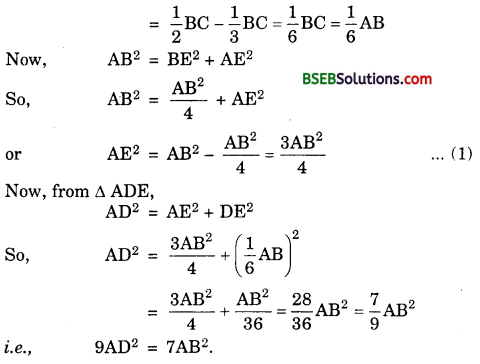Question 16.
In an equilateral triangle, prove that three times the square of one side is equal to four times the square of one of its altitudes.
Solution:
Let ABC be an equilateral triangle and let AD ⊥ BC. In ∆ ADB and ∆ ADC, we have :
AB = AC [Given]
By RHS criterion of congruence, we have :
So, BD = DC or BD = DC = 12BC … (1)
Since ∆ ADB is a right triangle, right angled at D, by Pythagoras theorem, we have :Hence, the result.

Question 17.
Tick the correct answer and justify :
In ∆ ABC, AB = 63‾√cm, AC = 12 cm and BC = 6 cm. The angle B is :
(A) 120°
(B) 60°
(C) 90°
(D) 45°
Solution:
In ∆ ABC, we have :AB = 63‾√ cm, AC = 12 cm
and BC = 6 cm … (1)
Now, AB² + BC²= (63‾√)² + (6)² [From (1)]
= 36 x 3 + 36
= 108 + 36 = 144
= (AC)²
∴ Thus, ∆ ABC is a right triangle, right angled at B.
∠B = 90°
∴ (C) is the correct choice.

## Bihar Board Class 10th Maths Chapter 6 Triangles Ex 6.5 Textbooks for Exam Preparations

Bihar Board Class 10th Maths Chapter 6 Triangles Ex 6.5 Textbook Solutions can be of great help in your Bihar Board Class 10th Maths Chapter 6 Triangles Ex 6.5 exam preparation. The BSEB STD 10th Maths Chapter 6 Triangles Ex 6.5 Textbooks study material, used with the English medium textbooks, can help you complete the entire Class 10th Maths Chapter 6 Triangles Ex 6.5 Books State Board syllabus with maximum efficiency.

## FAQs Regarding Bihar Board Class 10th Maths Chapter 6 Triangles Ex 6.5 Textbook Solutions

#### Can we get a Bihar Board Book PDF for all Classes?

Yes you can get Bihar Board Text Book PDF for all classes using the links provided in the above article.

## Important Terms

Bihar Board Class 10th Maths Chapter 6 Triangles Ex 6.5, BSEB Class 10th Maths Chapter 6 Triangles Ex 6.5 Textbooks, Bihar Board Class 10th Maths Chapter 6 Triangles Ex 6.5, Bihar Board Class 10th Maths Chapter 6 Triangles Ex 6.5 Textbook solutions, BSEB Class 10th Maths Chapter 6 Triangles Ex 6.5 Textbooks Solutions, Bihar Board STD 10th Maths Chapter 6 Triangles Ex 6.5, BSEB STD 10th Maths Chapter 6 Triangles Ex 6.5 Textbooks, Bihar Board STD 10th Maths Chapter 6 Triangles Ex 6.5, Bihar Board STD 10th Maths Chapter 6 Triangles Ex 6.5 Textbook solutions, BSEB STD 10th Maths Chapter 6 Triangles Ex 6.5 Textbooks Solutions,
Share: